# Simple Machine

#### Machine

Machine is any device by means of which a force applied at one point can be used to overcome a force at a different point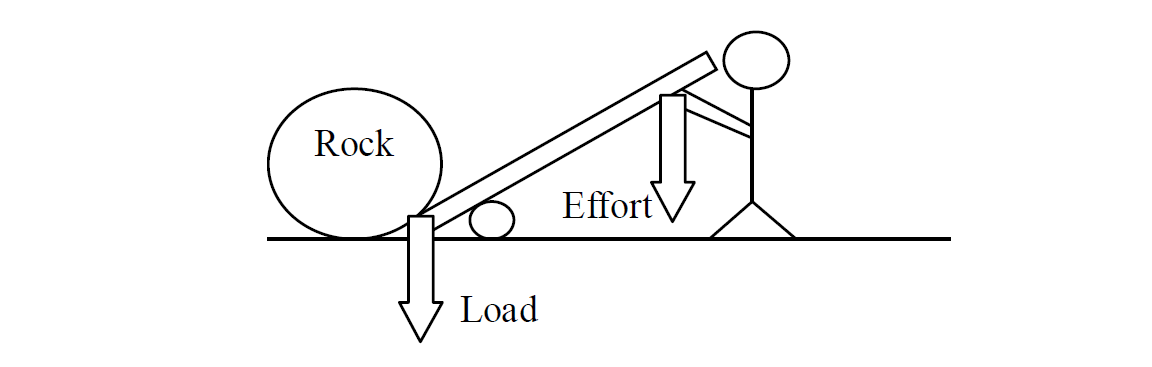The applied force is called Effort

The force which effort overcomes is called Load (The force which an object pulls or pushes on a machine is called Load.)

#### Types of simple machine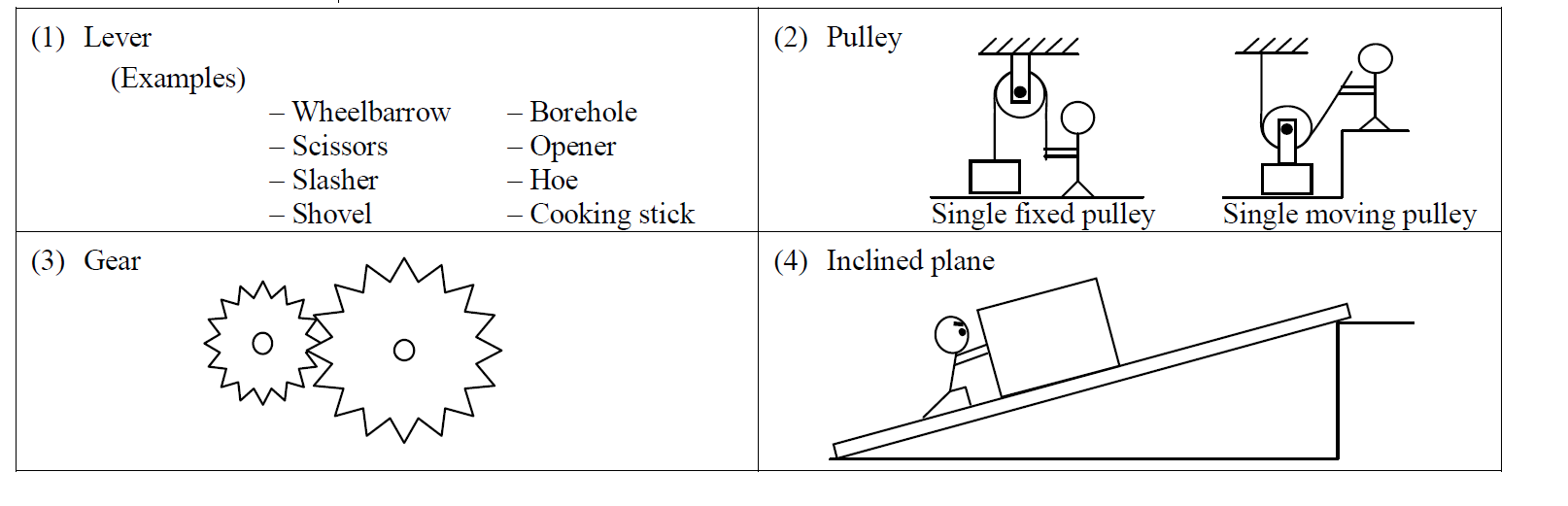The mechanical advantage of a machine is defined as the ratio of the load to the effort.

$\mathrm{M.A}=\frac{\mathrm{load}}{Effort}$

#### Velocity Ratio (V.R.)

The velocity ratio of a machine is defined as the ratio of the distance moved by effort to the distance moved by the load in the same time.

Formula for Velocity Ratio (V.R.)

$\mathrm{V.R}=\frac{\mathrm{distance moved by effort\left(dE\right)}}{distance moved by load in the same time\left(dL\right)}$

#### Efficiency

The efficiency of a machine is defined as the ratio of the useful work done by the machine to the total work put into the machine.

Formula for Efficiency

$\mathrm{Efficiency}=\frac{\mathrm{M.A}}{V.R}×100%$

#### Example 1

Calculate the mechanical advantage of the diagrams below.

1.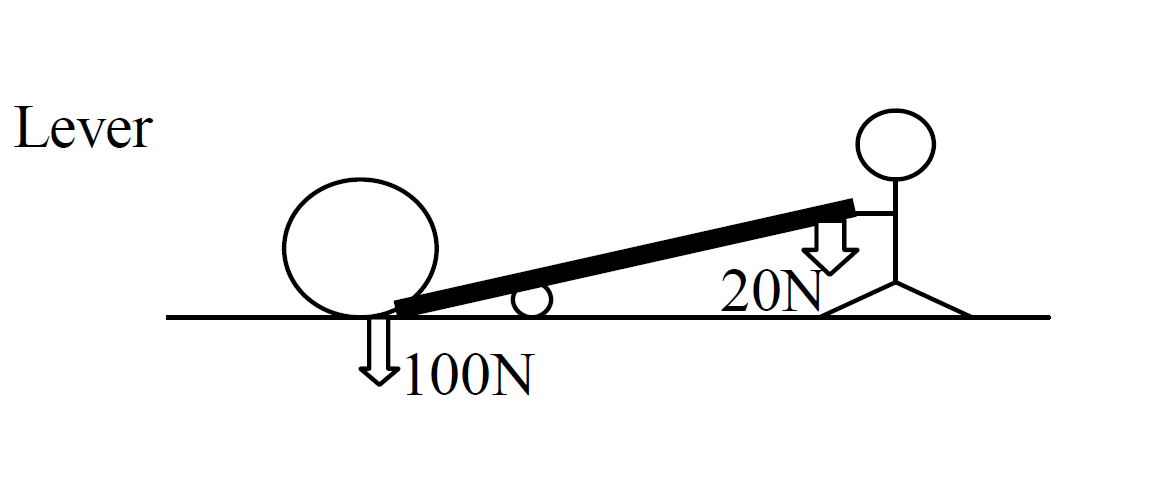Solution

$\mathrm{M.A}=\frac{\mathrm{load}}{Effort}$

given that

Effort = 20N

M.A = ?

$\mathrm{M.A}=\frac{\mathrm{100}}{20}$

Answer: $\mathrm{M.A}=5$

2.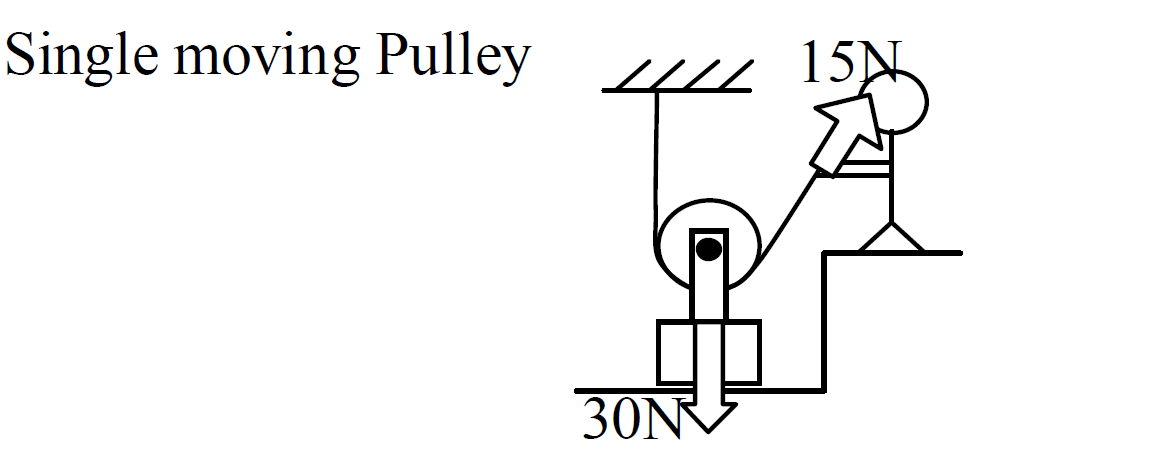Solution

$\mathrm{M.A}=\frac{\mathrm{load}}{Effort}$

given that

Effort = 15N

M.A = ?

$\mathrm{M.A}=\frac{\mathrm{30}}{15}$

Answer: $\mathrm{M.A}=2$

3.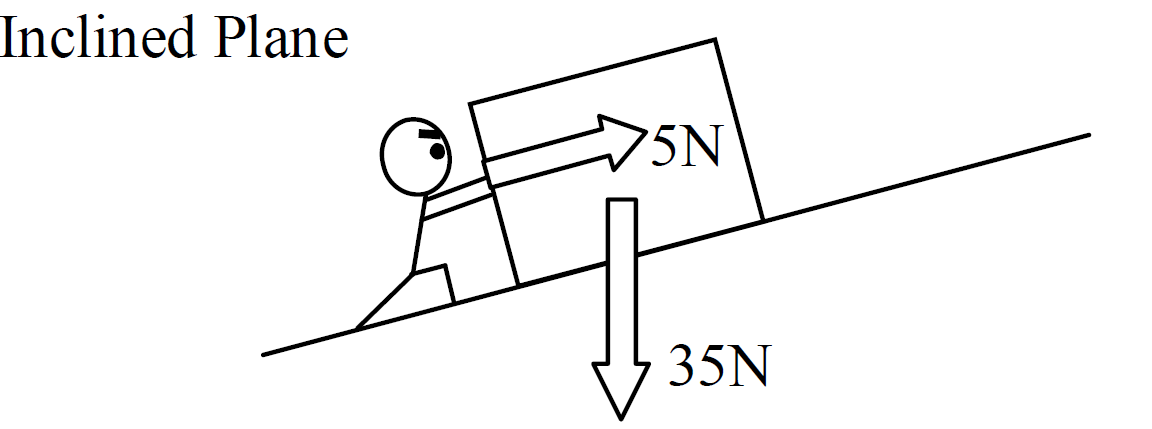Solution

$\mathrm{M.A}=\frac{\mathrm{load}}{Effort}$

given that

Effort = 5N

M.A = ?

$\mathrm{M.A}=\frac{\mathrm{35}}{5}$

Answer: $\mathrm{M.A}=7$

#### Example 2

Calculate the velocity ratio of the diagrams below.

1.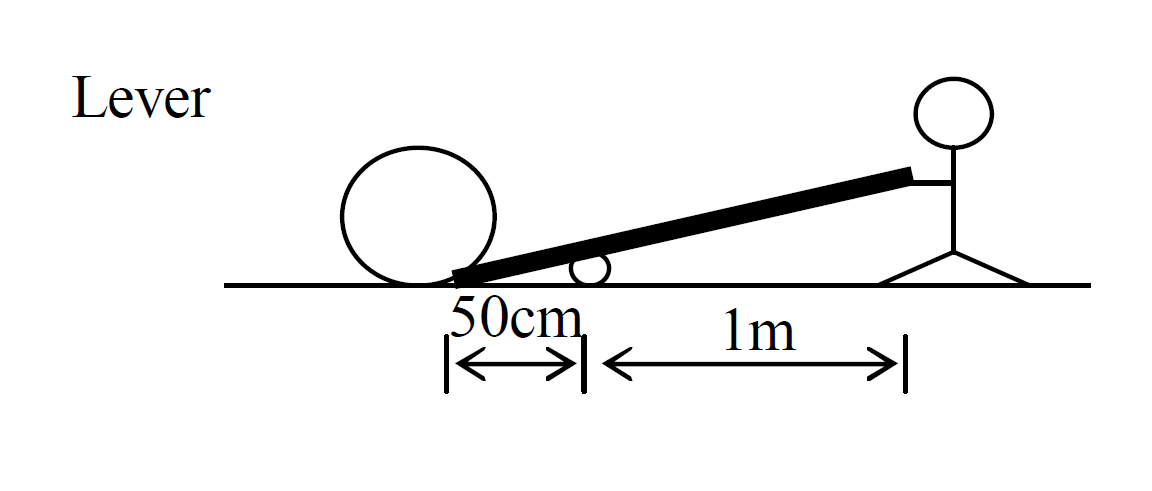Solution

$\mathrm{V.R}=\frac{\mathrm{distance moved by effort\left(dE\right)}}{distance moved by load in the same time\left(dL\right)}$

Given that

dE = 1m

dL = 50cm

V.R = ?

First convert 50cm to metres(m)

dL = 0.5m

$\mathrm{V.R}=\frac{1}{0.5}$

Answer: $\mathrm{V.R}=2$

#### Example 3

The diagram below shows a pulley system. An effort of 1000N is required to raise a load of 4500N.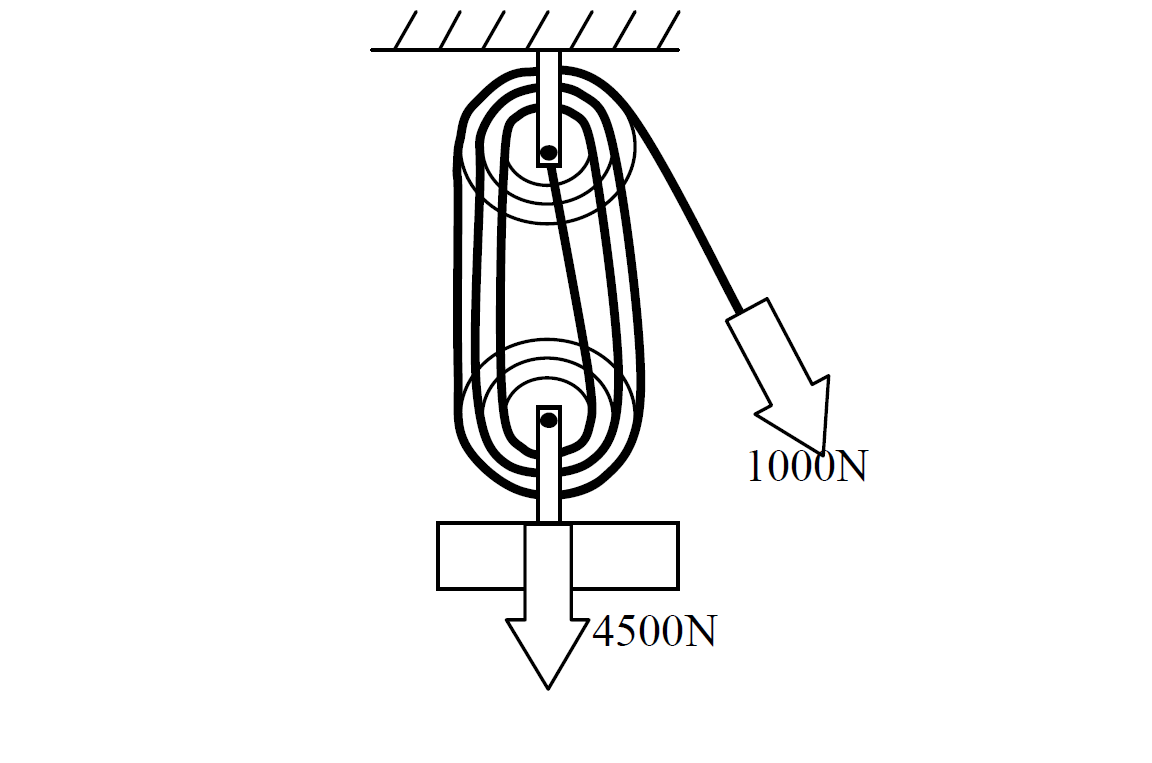(b) Find the velocity ratio.

(c) Find the efficiency.

Solution

(a)

$\mathrm{M.A}=\frac{\mathrm{load}}{Effort}$

Given that

M.A = ?

Effort = 1000N

$\mathrm{M.A}=\frac{\mathrm{4500}}{1000}$

Answer: $\mathrm{M.A}=\mathrm{4.5}$

(b)

To find the velocity ratio of the pulley system, count the number of lines connected to moving pulley.

Therefore 6 lines connected to moving pulleys.

(c)

$\mathrm{Efficiency}=\frac{\mathrm{M.A}}{V.R}×100%$

Efficiency = ?

M.A = 4.5

V.R = 6

$\mathrm{Efficiency}=\frac{\mathrm{4.5}}{6}×100%$

Answer: $\mathrm{Efficiency}=\mathrm{75%}$